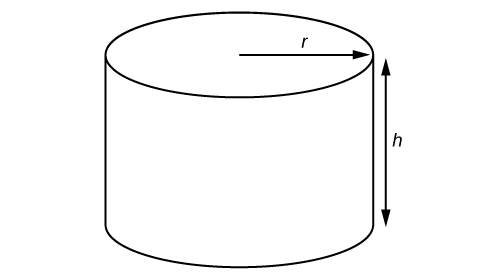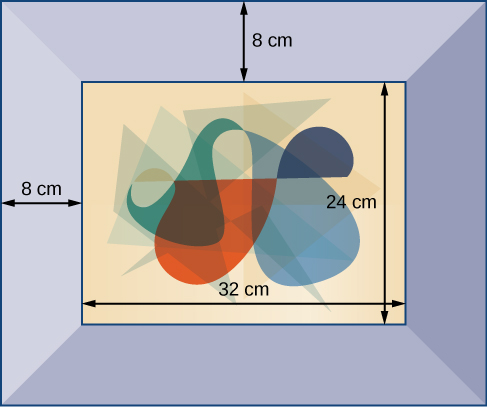## Evaluate and Simplify Algebraic Expressions

### Learning Outcomes

• List the constants and variables in an algebraic expression.
• Evaluate an algebraic expression.
• Use an algebraic formula.

So far, the mathematical expressions we have seen have involved real numbers only. In mathematics, we may see expressions such as $x+5,\frac{4}{3}\pi {r}^{3}$, or $\sqrt{2{m}^{3}{n}^{2}}$. In the expression $x+5, 5$ is called a constant because it does not vary and x is called a variable because it does. (In naming the variable, ignore any exponents or radicals containing the variable.) An algebraic expression is a collection of constants and variables joined together by the algebraic operations of addition, subtraction, multiplication, and division.

We have already seen some real number examples of exponential notation, a shorthand method of writing products of the same factor. When variables are used, the constants and variables are treated the same way.

\begin{align}&\left(-3\right)^{5}=\left(-3\right)\cdot\left(-3\right)\cdot\left(-3\right)\cdot\left(-3\right)\cdot\left(-3\right) && x^{5}=x\cdot x\cdot x\cdot x\cdot x \\ &\left(2\cdot7\right)^{3}=\left(2\cdot7\right)\cdot\left(2\cdot7\right)\cdot\left(2\cdot7\right) && \left(yz\right)^{3}=\left(yz\right)\cdot\left(yz\right)\cdot\left(yz\right)\\ \text{ }\end{align}

In each case, the exponent tells us how many factors of the base to use, whether the base consists of constants or variables.

Any variable in an algebraic expression may take on or be assigned different values. When that happens, the value of the algebraic expression changes. To evaluate an algebraic expression means to determine the value of the expression for a given value of each variable in the expression. Replace each variable in the expression with the given value, then simplify the resulting expression using the order of operations. If the algebraic expression contains more than one variable, replace each variable with its assigned value and simplify the expression as before.

### Example: Describing Algebraic Expressions

List the constants and variables for each algebraic expression.

1. x + 5
2. $\frac{4}{3}\pi {r}^{3}$
3. $\sqrt{2{m}^{3}{n}^{2}}$

### Example: Evaluating an Algebraic Expression at Different Values

Evaluate the expression $2x - 7$ for each value for x.

1. $x=0$
2. $x=1$
3. $x=\dfrac{1}{2}$
4. $x=-4$

### Example: Evaluating Algebraic Expressions

Evaluate each expression for the given values.

1. $x+5$ for $x=-5$
2. $\frac{t}{2t - 1}$ for $t=10$
3. $\dfrac{4}{3}\pi {r}^{3}$ for $r=5$
4. $a+ab+b$ for $a=11,b=-8$
5. $\sqrt{2{m}^{3}{n}^{2}}$ for $m=2,n=3$

### Try It

In the following video we present more examples of how to evaluate an expression for a given value.

## Formulas

An equation is a mathematical statement indicating that two expressions are equal. The expressions can be numerical or algebraic. The equation is not inherently true or false, but only a proposition. The values that make the equation true, the solutions, are found using the properties of real numbers and other results. For example, the equation $2x+1=7$ has the unique solution $x=3$ because when we substitute 3 for $x$ in the equation, we obtain the true statement $2\left(3\right)+1=7$.

A formula is an equation expressing a relationship between constant and variable quantities. Very often the equation is a means of finding the value of one quantity (often a single variable) in terms of another or other quantities. One of the most common examples is the formula for finding the area $A$ of a circle in terms of the radius $r$ of the circle: $A=\pi {r}^{2}$. For any value of $r$, the area $A$ can be found by evaluating the expression $\pi {r}^{2}$.

### Example: Using a Formula

A right circular cylinder with radius $r$ and height $h$ has the surface area $S$ (in square units) given by the formula $S=2\pi r\left(r+h\right)$. Find the surface area of a cylinder with radius 6 in. and height 9 in. Leave the answer in terms of $\pi$.Right circular cylinder

### Try ItFigure 4

A photograph with length L and width W is placed in a matte of width 8 centimeters (cm). The area of the matte (in square centimeters, or cm2) is found to be $A=\left(L+16\right)\left(W+16\right)-L\cdot W$. Find the area of a matte for a photograph with length 32 cm and width 24 cm.

## Simplify Algebraic Expressions

Sometimes we can simplify an algebraic expression to make it easier to evaluate or to use in some other way. To do so, we use the properties of real numbers. We can use the same properties in formulas because they contain algebraic expressions.

### Example: Simplifying Algebraic Expressions

Simplify each algebraic expression.

1. $3x - 2y+x - 3y - 7$
2. $2r - 5\left(3-r\right)+4$
3. $\left(4t-\dfrac{5}{4}s\right)-\left(\dfrac{2}{3}t+2s\right)$
4. $2mn - 5m+3mn+n$

### Example: Simplifying a Formula

A rectangle with length $L$ and width $W$ has a perimeter $P$ given by $P=L+W+L+W$. Simplify this expression.

### Try It

If the amount $P$ is deposited into an account paying simple interest $r$ for time $t$, the total value of the deposit $A$ is given by $A=P+Prt$. Simplify the expression. (This formula will be explored in more detail later in the course.)

## Contribute!

Did you have an idea for improving this content? We’d love your input.